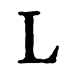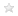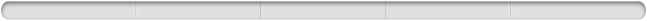Aquest lloc utilitza galetes per a oferir els nostres serveis, millorar el desenvolupament, per a anàlisis i (si no has iniciat la sessió) per a publicitat. Utilitzant LibraryThing acceptes que has llegit i entès els nostres Termes de servei i política de privacitat. L'ús que facis del lloc i dels seus serveis està subjecte a aquestes polítiques i termes.
Hide this

Clica una miniatura per anar a Google Books.

S'està carregant…

# Structure of algebras (edició 1939)

 Membres Ressenyes Popularitat Valoració mitjana Converses 13 Cap 1,306,155 Cap Cap The first three chapters of this work contain an exposition of the Wedderburn structure theorems. Chapter IV contains the theory of the commutator subalgebra of a simple subalgebra of a normal simple algebra, the study of automorphisms of a simple algebra, splitting fields, and the index reduction factor theory. The fifth chapter contains the foundation of the theory of crossed products and of their special case, cyclic algebras. The theory of exponents is derived there as well as the consequent factorization of normal division algebras into direct factors of prime-power degree. Chapter VI consists of the study of the abelian group of cyclic systems which is applied in Chapter VII to yield the theory of the structure of direct products of cyclic algebras and the consequent properties of norms in cyclic fields. This chapter is closed with the theory of \$p\$-algebras. In Chapter VIII an exposition is given of the theory of the representations of algebras. The treatment is somewhat novel in that while the recent expositions have used representation theorems to obtain a number of results on algebras, here the theorems on algebras are themselves used in the derivation of results on representations. The presentation has its inspiration in the author's work on the theory of Riemann matrices and is concluded by the introduction to the generalization (by H. Weyl and the author) of that theory. The theory of involutorial simple algebras is derived in Chapter X both for algebras over general fields and over the rational field. The results are also applied in the determination of the structure of the multiplication algebras of all generalized Riemann matrices, a result which is seen in Chapter XI to imply a complete solution of the principal problem on Riemann matrices.… (més)
 Membre: morphismus Títol: Structure of algebras Autors: A. Adrian Albert Informació: New York, American Mathematical Society, 1939. Col·leccions: La teva biblioteca Valoració:Etiquetes: Cap

#### Informació de l'obra

Structure of Algebras de A. Adrian Albert

 Afegit fa poc per konyv, morphismus, BYUMath, wilkesmcs, differentia, dominicsoda, hmcmath, appleby, JohnCernes
Cap
S'està carregant…Apunta't a LibraryThing per saber si aquest llibre et pot agradar.

No hi ha cap discussió a Converses sobre aquesta obra.

Sense ressenyes
Has d'iniciar sessió per poder modificar les dades del coneixement compartit.
Si et cal més ajuda, mira la pàgina d'ajuda del coneixement compartit.
Títol normalitzatTítol originalTítols alternatiusData original de publicacióGent/PersonatgesLlocs importantsEsdeveniments importantsPremis i honorsEpígrafDedicatòriaPrimeres paraulesCitacionsDarreres paraulesNota de desambiguacióEditor de l'editorialCreadors de notes promocionals a la cobertaLlengua originalCDD/SMD canònicsLCC canònicReferències a aquesta obra en fonts externes.

##Wikipedia en anglès (3)

 Abraham Adrian AlbertNilpotent algebra Non-associative algebra
The first three chapters of this work contain an exposition of the Wedderburn structure theorems. Chapter IV contains the theory of the commutator subalgebra of a simple subalgebra of a normal simple algebra, the study of automorphisms of a simple algebra, splitting fields, and the index reduction factor theory. The fifth chapter contains the foundation of the theory of crossed products and of their special case, cyclic algebras. The theory of exponents is derived there as well as the consequent factorization of normal division algebras into direct factors of prime-power degree. Chapter VI consists of the study of the abelian group of cyclic systems which is applied in Chapter VII to yield the theory of the structure of direct products of cyclic algebras and the consequent properties of norms in cyclic fields. This chapter is closed with the theory of \$p\$-algebras. In Chapter VIII an exposition is given of the theory of the representations of algebras. The treatment is somewhat novel in that while the recent expositions have used representation theorems to obtain a number of results on algebras, here the theorems on algebras are themselves used in the derivation of results on representations. The presentation has its inspiration in the author's work on the theory of Riemann matrices and is concluded by the introduction to the generalization (by H. Weyl and the author) of that theory. The theory of involutorial simple algebras is derived in Chapter X both for algebras over general fields and over the rational field. The results are also applied in the determination of the structure of the multiplication algebras of all generalized Riemann matrices, a result which is seen in Chapter XI to imply a complete solution of the principal problem on Riemann matrices.

No s'han trobat descripcions de biblioteca.

Descripció del llibreSumari haiku## Classificació Decimal de Dewey (DDC)

512.24 — Natural sciences and mathematics Mathematics Algebra Groups and groups theory Imaginary expressions

## Valoració

Mitjana: Sense puntuar.

## Ets tu?

Fes-te Autor del LibraryThing.

Quant a | Contacte | LibraryThing.com | Privadesa/Condicions | Ajuda/PMF | Blog | Botiga | APIs | TinyCat | Biblioteques llegades | Crítics Matiners | Coneixement comú | 170,073,101 llibres! | Barra superior: Sempre visible